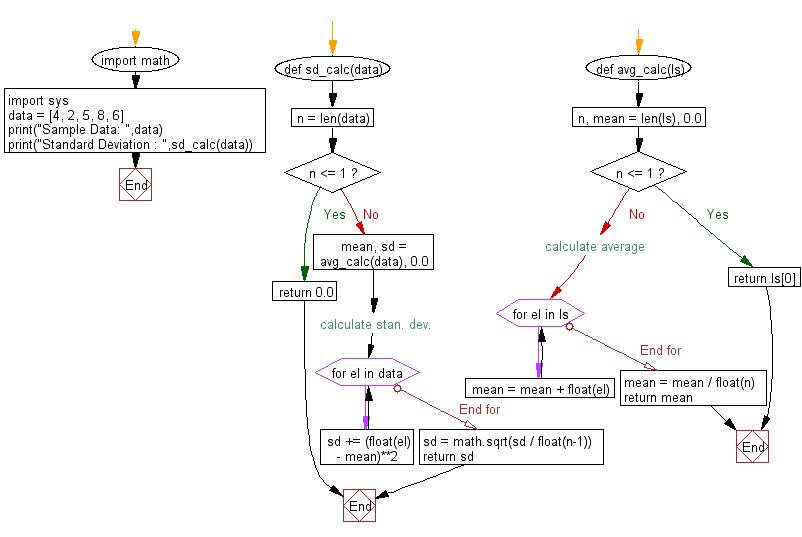﻿ Python Math: Calculate the standard deviation - w3resource# Python Math: Calculate the standard deviation

## Python Math: Exercise-57 with Solution

Write a Python program to calculate the standard deviation of the following data.

Sample Solution:-

Python Code:

``````import math
import sys

def sd_calc(data):
n = len(data)

if n <= 1:
return 0.0

mean, sd = avg_calc(data), 0.0

# calculate stan. dev.
for el in data:
sd += (float(el) - mean)**2
sd = math.sqrt(sd / float(n-1))

return sd

def avg_calc(ls):
n, mean = len(ls), 0.0

if n <= 1:
return ls

# calculate average
for el in ls:
mean = mean + float(el)
mean = mean / float(n)

return mean

data = [4, 2, 5, 8, 6]
print("Sample Data: ",data)
print("Standard Deviation : ",sd_calc(data))
```
```

Sample Output:

```Sample Data:  [4, 2, 5, 8, 6]
Standard Deviation :  2.23606797749979
```

Pictorial Presentation:Flowchart:Python Code Editor:

Have another way to solve this solution? Contribute your code (and comments) through Disqus.

What is the difficulty level of this exercise?

Test your Programming skills with w3resource's quiz.

﻿

```>>> students = [{'name': 'John', 'score': 98}, {'name': 'Mike', 'score': 94}, {'name': 'Jennifer', 'score': 99}]